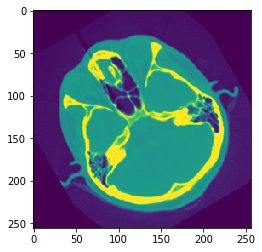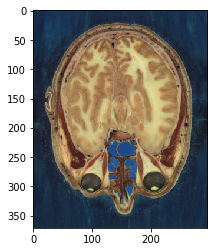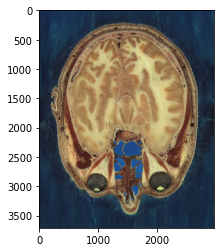# Using matplotlib to display inline images¶

In this notebook we will explore using matplotlib to display images in our notebooks, and work towards developing a reusable function to display 2D,3D, color, and label overlays for SimpleITK images.

We will also look at the subtleties of working with image filters that require the input images' to be overlapping.

In :
%matplotlib inline
import matplotlib.pyplot as plt
import SimpleITK as sitk


SimpleITK has a built in Show method which saves the image to disk and launches a user configurable program ( defaults to ImageJ ), to display the image.

In :
img1 = sitk.ReadImage(fdata("cthead1.png"))

Fetching cthead1.png

In :
img2 = sitk.ReadImage(fdata("VM1111Shrink-RGB.png"))

Fetching VM1111Shrink-RGB.png

In :
nda = sitk.GetArrayViewFromImage(img1)
plt.imshow(nda)

Out:
<matplotlib.image.AxesImage at 0x10767aa90>In :
nda = sitk.GetArrayViewFromImage(img2)
ax = plt.imshow(nda)In :
def myshow(img):
nda = sitk.GetArrayViewFromImage(img)
plt.imshow(nda)

In :
myshow(img2)In :
myshow(sitk.Expand(img2, *5))This image does not appear bigger.

There are numerous improvements that we can make:

• support 3d images
• include a title
• use physical pixel size for axis labels
• show the image as gray values
In :
def myshow(img, title=None, margin=0.05, dpi=80):
nda = sitk.GetArrayViewFromImage(img)
spacing = img.GetSpacing()

if nda.ndim == 3:
# fastest dim, either component or x
c = nda.shape[-1]

# the the number of components is 3 or 4 consider it an RGB image
if not c in (3,4):
nda = nda[nda.shape//2,:,:]

elif nda.ndim == 4:
c = nda.shape[-1]

if not c in (3,4):
raise Runtime("Unable to show 3D-vector Image")

# take a z-slice
nda = nda[nda.shape//2,:,:,:]

ysize = nda.shape
xsize = nda.shape

# Make a figure big enough to accommodate an axis of xpixels by ypixels
# as well as the ticklabels, etc...
figsize = (1 + margin) * ysize / dpi, (1 + margin) * xsize / dpi

fig = plt.figure(figsize=figsize, dpi=dpi)
# Make the axis the right size...
ax = fig.add_axes([margin, margin, 1 - 2*margin, 1 - 2*margin])

extent = (0, xsize*spacing, ysize*spacing, 0)

t = ax.imshow(nda,extent=extent,interpolation=None)

if nda.ndim == 2:
t.set_cmap("gray")

if(title):
plt.title(title)

In :
myshow(sitk.Expand(img2,[2,2]), title="Big Visibile Human Head")